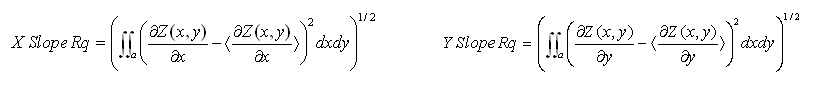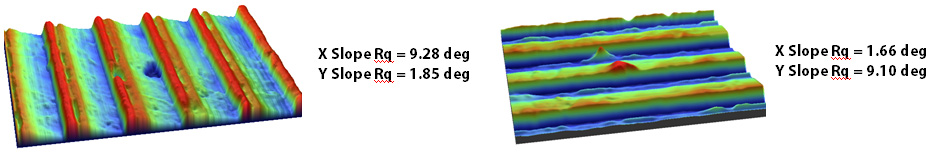3D Surface Roughness and Wear Measurement, Analysis and Inspection

# Root Mean Square Slope in X,Y

### Slope Rq (X,Y)

X Slope Rq and Y Slope Rq, the Root Mean Squared X Slope and Root Mean Squared Y Slope are found by calculating the standard deviation (i.e. rms) of the slopes of the surface along the X and Y directions respectively. The slope is found by taking the derivative of the surface profiles along each direction, using the lateral resolution of the measurement area as the point spacing. Analytically, X Slope Rq and Y Slope Rq are given by:Where the brackets, < >, represent the average value of all slopes in the relevant direction.### Application

Slopes may be used to investigate the behavior of various surface texture forming techniques in that material and process conditions may change the slopes considerably, whereas the height parameters such as Ra are relatively unaffected. The surface slope may also be useful in relating the nature of the surface when used in coating and visual appearance applications.